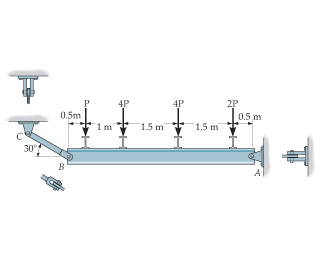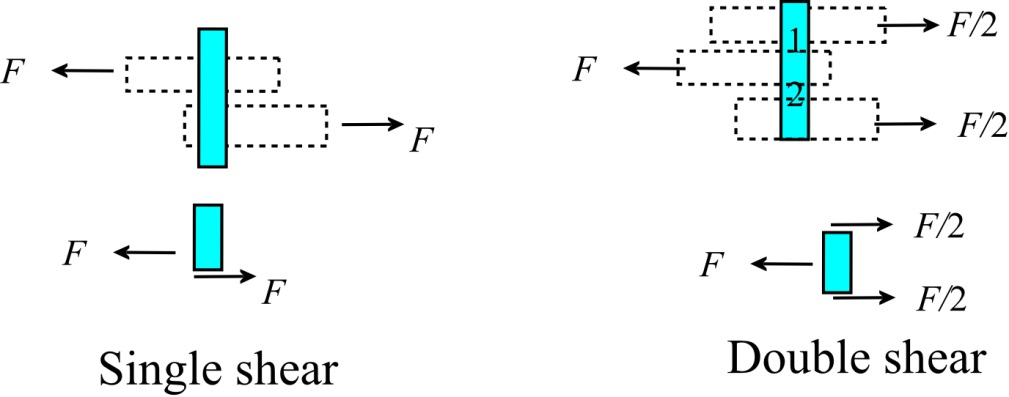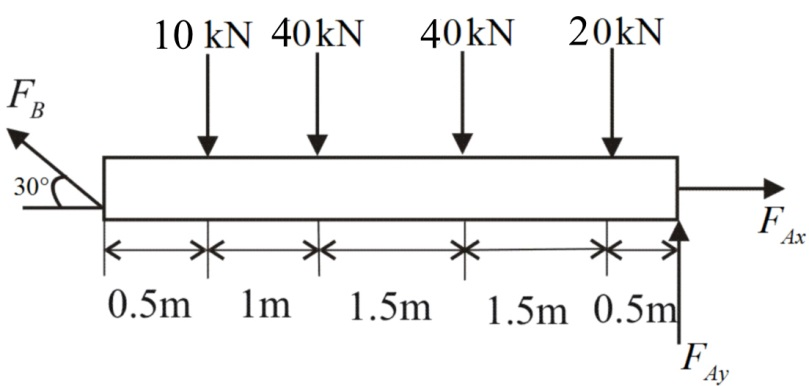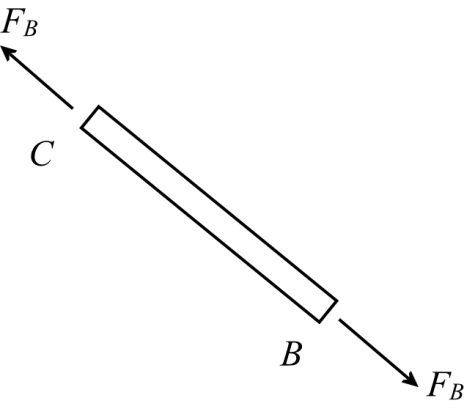In: Other

# The beam is supported by a pin at A and a short link BC. If P = 10kN , determine the average shear stress developed...

The beam is supported by a pin at A and a short link BC.

If P = 10kN, determine the average shear stress developed in the pins atA, B, and C . All pins are in double shear as shown, and each has a diameter of 17mm## Solutions

##### Expert Solution

Concepts and reason

A body is in equilibrium if vector sum of all the forces is equal to zero or moment of all force vectors about any point is equal to zero.

Magnitude of moment can be calculated by multiplying the magnitude of force with the perpendicular distance between the point where the force is applied and the point where the moment to be found.

Shear stress:

It is the ratio of a force and area tangential to the action of force.

Double shear stress:

Consider a pin on which two supports are connected.Here, two shear stress acts on the pin at location 1 and 2. Shear 1 on pin due to left support and top right support, shear 2 due to left support and bottom right support.

So double shear is given as follows:

${\left( \tau \right)_{avg}} = \frac{F}{{2A}}$

Fundamentals

Write the equilibrium equation of force vector.

$\begin{array}{l}\\\sum {{\bf{F}} = 0} \\\\{{\bf{F}}_1} + {{\bf{F}}_2} + .... + {{\bf{F}}_n} = 0\\\end{array}$

Moment of can be calculated as follows:

It can be calculated by multiplying the magnitude of force with the distance between the point of application of force and point about which moment is to be calculated.

General sign convection for moment: The moment is considered positive in counter-clockwise direction and negative in clockwise direction.

General sign convection for axis: Distance along the axis is positive and opposite to the axis is negative.

Draw the free body diagram of the beam BA.$\begin{array}{l}\\\sum {{M_A} = 0} \\\\ - \left( {{F_B}\sin 30^\circ \times 5} \right) + \left( {10 \times 4.5} \right) + \left( {40 \times 3.5} \right) + \left( {40 \times 2} \right) + \left( {20 \times 0.5} \right) = 0\\\\{F_B} = 110{\rm{ kN}}\\\end{array}$

Apply equilibrium conditions along x axis.

$\begin{array}{l}\\\sum {{F_x} = 0} \\\\{F_{Ax}} - 110\cos 30^\circ = 0\\\\{F_{Ax}} = 95.26{\rm{ kN}}\\\end{array}$

Apply equilibrium conditions along y axis.

$\begin{array}{l}\\\sum {{F_y} = 0} \\\\{F_{Ay}} + 110\sin 30^\circ - 10 - 40 - 40 - 20 = 0\\\\{F_{Ay}} = 55{\rm{ kN}}\\\end{array}$

Determine the total reaction force at pin A.

${F_A} = \sqrt {{{\left( {{F_{Ax}}} \right)}^2} + {{\left( {{F_{Ay}}} \right)}^2}}$

Substitute $55{\rm{ kN}}$ for ${F_{Ay}}$ , and $95.26{\rm{ kN}}$ for ${F_{Ax}}$ .

$\begin{array}{c}\\{F_A} = \sqrt {{{\left( {{F_{Ax}}} \right)}^2} + {{\left( {{F_{Ay}}} \right)}^2}} \\\\ = \sqrt {{{\left( {55} \right)}^2} + {{\left( {95.26} \right)}^2}} \\\\ = 110\;{\rm{kN}}\\\end{array}$

Consider the free body diagram of the link BC and pins at A, B and C.Consider the free body diagram of link B and write equilibrium of forces along the link.

${F_B} = 110{\rm{ kN}}$

Calculate the shear stress in the pins due to double shear.

$\begin{array}{c}\\{\left( {{\tau _B}} \right)_{avg}} = \frac{{{F_B}}}{{2 \times {A_C}}}\\\\ = \frac{{{F_B}}}{{2 \times \frac{\pi }{4}{D^2}}}\\\end{array}$

Here, diameter of the pin is D.

Substitute 17mm for D and $110{\rm{ kN}}$ for ${F_B}$ .

$\begin{array}{c}\\{\left( {{\tau _B}} \right)_{avg}} = \frac{{110 \times {{10}^3}}}{{2 \times \frac{\pi }{4} \times {{\left( {17} \right)}^2}}}\\\\ = 242.312\,{\rm{N/m}}{{\rm{m}}^2}\\\end{array}$

Consider force equilibrium in link BC.

$\begin{array}{l}\\{F_B} = 110{\rm{ kN}}\\\\{F_C} = 110{\rm{ kN}}\\\end{array}$

Calculate the shear stress in the pins due to double shear.

$\begin{array}{c}\\{\left( {{\tau _C}} \right)_{avg}} = \frac{{{F_C}}}{{2 \times {A_C}}}\\\\ = \frac{{{F_C}}}{{2 \times \frac{\pi }{4}{D^2}}}\\\end{array}$

Substitute 17mm for D and $110{\rm{ kN}}$ for ${F_C}$ .

$\begin{array}{c}\\{\left( {{\tau _C}} \right)_{avg}} = \frac{{110 \times {{10}^3}}}{{2 \times \frac{\pi }{4} \times {{17}^2}}}\\\\ = 242.312\,{\rm{N/m}}{{\rm{m}}^2}\\\end{array}$

Calculate the average shear stress in pin at A.

${\left( {{\tau _A}} \right)_{avg}} = \frac{{{F_A}}}{{2 \times {A_A}}}$

Substitute 17mm for D and $110{\rm{ kN}}$ for ${F_A}$ .

$\begin{array}{c}\\{\left( {{\tau _A}} \right)_{avg}} = \frac{{110 \times {{10}^3}}}{{2 \times \frac{\pi }{4} \times {{17}^2}}}\\\\ = 242.312\,{\rm{N/m}}{{\rm{m}}^2}\\\end{array}$

Ans:

The average shear stress in the pin at point B is $242.312\,{\rm{N/m}}{{\rm{m}}^2}$ .

## Related Solutions

##### Determine the required shear reinforcing for a simply supported concrete beam 7 meters long with a...
Determine the required shear reinforcing for a simply supported concrete beam 7 meters long with a cross section of 500 millimeters in depth and a width of 380 millimeters. Use fc = 35 MPa fy = 400 MPa. The longitudinal steel uses one row of 25M bars. The specific live load is 29 kKn/m. The beam is not exposed.
##### Draw the enveloped shear diagram and enveloped moment diagram for a simply supported beam with a...
Draw the enveloped shear diagram and enveloped moment diagram for a simply supported beam with a uniform dead load of 1.00kip/ft, a uniform live load of 1.50kip/ft and a concentrated live load of 175 kip. the dead load is applied to te entire span. the live load may be positioned anywhere on the span.
##### Determine the shear stress developed on a 3-m circular shaft with D=250 mm, G = 83GPa...
Determine the shear stress developed on a 3-m circular shaft with D=250 mm, G = 83GPa applied with the torque, T = 100 kN-m, a. at the center of the cross-section b. at a point 50 mm away from the center c. at a point 100 mm away from the center d. maximum distance from the center
##### The allowable normal stress for member BC is σallow = 29 ksi and the allowable shear stress for the pins is τallow = 10 ksi.
The allowable normal stress for member BC is σallow = 29 ksi and the allowable shear stress for the pins is τallow = 10 ksi.(Figure 1)FigurePart ADetermine the required thickness of member BC.Express your answer to three significant figures and include the appropriate units.t =Part BDetermine the required diameter of the pin at A.Express your answer to three significant figures and include the appropriate units.dA =Part CDetermine the required diameter of the pin at B.Express your answer to three significant figures and include the appropriate units.dB =
##### The beam is constructed from three boards. (Figure 1) The allowable shear stress for the wood is τallow=335 psi. Each nail can resist a shear force of 395 lb. Part A Determine the maximum loads P tha...
The beam is constructed from three boards. (Figure 1) The allowable shear stress for the wood is τallow=335 psi. Each nail can resist a shear force of 395 lb.Part ADetermine the maximum loads P that it can support.Express your answer to three significant figures and include appropriate units.Part BWhat is the maximum allowable spacing s of the nails used to hold the top flange to the web? (Consider only regions AC and BD.)Express your answer to three significant figures and...
##### Determine the force developed in members FE, FC and BC of the truss and state if...
Determine the force developed in members FE, FC and BC of the truss and state if these members are in tension or compression. Given, Pin support at A and Roller support at D. Use Section Method.
##### Determine the critical resolved shear stress for an iron crystal which deforms by simultaneous slip on...
Determine the critical resolved shear stress for an iron crystal which deforms by simultaneous slip on (110) , (110) , (110), and (110)  when the tensile stress along  is 95.2 MPa. {Manual calculations perfectly OK. But it would be good to do this on a spreadsheet.}
##### Assume if the shear stress in steel exceeds about 4.00x108 N/m2 the steel   ruptures. (a) Determine...
Assume if the shear stress in steel exceeds about 4.00x108 N/m2 the steel   ruptures. (a) Determine the shearing force necessary to shear a steel bolt 1.25 cm in diameter. (b) Determine the shearing force necessary to punch a 1.60-cm-diameter hole in a steel plate 0.530 cm thick
##### Determine the location of the shear centre of the C-shaped rolled-steel beam cross-section C150x19.3 with respect...
Determine the location of the shear centre of the C-shaped rolled-steel beam cross-section C150x19.3 with respect to the centre of the web. Indicate whether it is to the left or right of the centre of the web. Assume the section to be thin walled and neglect the rounding of corners.